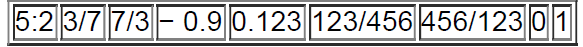×
Get Full Access to Elementary Statistics - 12 Edition - Chapter 4.2 - Problem 5bsc
Get Full Access to Elementary Statistics - 12 Edition - Chapter 4.2 - Problem 5bsc

×

# Identifying Probability Values Which of the followingISBN: 9780321836960 18

## Solution for problem 5BSC Chapter 4.2

Elementary Statistics | 12th Edition

• Textbook Solutions
• 2901 Step-by-step solutions solved by professors and subject experts
• Get 24/7 help from StudySoup virtual teaching assistantsElementary Statistics | 12th Edition

4 5 1 418 Reviews
21
1
Problem 5BSC

Identifying Probability Values Which of the following values are not probabilities?Step-by-Step Solution:
Step 1 of 3

Solution 5BSC

Principle: The probability of an event is a number (fraction or decimal) between 0 and 1 inclusive.

The valuecannot be a probability value because it is greater than 1.

The valuecan be a probability value because it is between 0 and 1.

The valuecannot be a probability value because it is greater than 1.

The valuecannot be a probability value because it is smaller than 0.

The valuecan be a probability value because it is between 0 and 1.

The valuecan be a probability value because it is between 0 and 1.

The valuecannot be a probability value because it is greater than 1.

The valuecan be a probability value because it is between 0 and 1 inclusive.

The valuecan be a probability value because it is between 0 and 1 inclusive.

Step 2 of 3

Step 3 of 3

##### ISBN: 9780321836960

Unlock Textbook Solution